## Gravitation Class 9 Important Questions and Answers Science Chapter 10

Based on gravitation and Newtons law of gravitation

Question 1.
What do you mean by gravitation ? (CBSE 2012)
The force of attraction between any two particles in the universe is called gravitation.

More Resources

Question 2.
State Universal law of gravitation.

Or

How is the force of attraction dependent on the masses of objects and distance between them ?
(CBSE 2014)
Each object on the universe attracts other object with a’ force known as gravitational force. The force of attraction between two objects is directly proportional to

1. the product of masses of the objects and
2. inversely proportional to the square of distance between them. That is,Question 3.
The distance between two particles is decreased. Will the gravitational force between them increase or decrease ?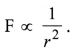So, gravitational force (F) increases when the distance (r) between two particles decreases. r

Question 4.
An iron ball exerts a force F on the rubber ball. How much force, the rubber ball will exert on the iron ball ?
The rubber ball also exerts the same force F on the iron ball.

Question 5.
Define Universal gravitational constant (G).
Universal gravitational constant is defined as the force of attraction between two objects of unit masses separated by unit distance.

Question 6.
Write down the unit of ‘G’ in S.I.
Nm2 kg-2.

Question 7.
What is the value of ‘G’ ?
G = 6.673 x 10-11 N m2 kg-2.

Question 8.
The value of G = 6.673 x 10-11 Nm2 kg-2 on the surface of the earth. What is the value of G on the surface of the moon ? (CBSE Sample Paper; CBSE 2011, 2013, 2014)
The value of G on the surface of the moon
= value of G on the surface of the earth (i.e., 6.673 x 10-11 Nm2 kg-2).

Question 9.
The gravitational force between two objects on the earth is 2 N. What will be the gravitational force between these two objects on the surface of the moon ?
Same force i.e. 2 N.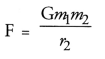(∴ F and G has the same value on the surface of earth and on the surface of moon).

Question 10.
State one phenomena related to universal law of gravitation.
The atmosphere of earth is possible due to this law.

Question 11.
Name the force which is responsible for the revolution of planets around the sun. (CBSE 2015)
The gravitational force of attraction between the planet and the sun. This force provides the necessary centripetal force to the planet to move in a circular orbit around the sun.

Question 12.
Name the force which is responsible for the revolution of moon around the earth.
The gravitational force between the earth and the moon provides the centripetal force to the moon to move in a circular path around the earth.

Question 13.
The earth is acted upon by the gravitational force of attraction due to the sun. Then why does the earth not fall towards the sun ?
Gravitational force of attraction on the earth due to the sun provides the necessary centripetal force to the earth to move around the earth. Hence, earth does not fall towards the sun.

Question 14.
State two factors on which gravitational force between two objects depends.

1. Mass of the objects,
2. distance between them.

Question 15.
The gravitational force between two objects is F. How will the force change when the distance between them is reduced to 1/4 th ?Question 16.
Is the force of gravity stronger on a piece of iron than on a piece of wood if both have the same mass ?
(CBSE 2015)
No. Force of gravity on a piece of iron is equal to the force of gravity on a piece of wood because F = mg.

Question 17.
When a stone is thrown vertically upwards, its velocity is continuously dedfeased. Why ? (CBSE 2015)
When a stone is thrown vertically upwards, a force called gravitational force pulls the stone continuously in the downward direction. Hence, velocity of the stone is decreased continuously.

Based on free fall and acceleration due to gravity

Question 18.
The factor associated with the motion of an object are : Force, velocity, acceleration and momentum. Out of these four factors which one remains constant for all bodies large or small, undergoing a free fall.
(CBSE 2013, 2015)
Acceleration of a freely falling object remains constant as it does not depend on the mass of the object.

Question 19.
Two objects of masses and M2 are dropped in vacuum from a height above the surface of earth (Mt> M2). Which one will fall the ground first, and why ?
Both objects will fall on the ground simultaneously as the acceleration (known as acceleration due to gravity) with which an object falls towards the earth is constant irrespective of the mass of the object.

Question 20.
When an object is dropped from a height, it accelerates when it falls down. Name the force which accelerates the object.
Gravitational force between object and earth (i.e., gravity).

Question 21.
State the type of motion shown by a freely falling stone. (CBSE 2015)
Uniformly accelerated motion.

Question 22.
Write the expression for the acceleration due to gravity. GMQuestion 23.
What is the SI unit of acceleration due to gravity (g) ?
m s-2.

Question 24.
What is the value of acceleration due to gravity on the surface of earth ?
g = 9.8 m s-2

Question 25.
What is the value of acceleration due to gravity on the surface of moon ?
g = 1.6 m s-2 on the surface of moon.

Question 26.
What is the direction of acceleration due to gravity ?
Towards the centre of the earth.

Question 27.
What will be the direction of acceleration due to gravity if the body is thrown vertically upward ?
The direction of acceleration due to gravity is always towards the centre of the earth i.e. in the downward direction.

Question 28.
Is value of ‘g’ same at all ‘places on the earth ? Give reason for your answer. (CBSE 2011)
No.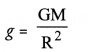Since polar radius (distance of pole from the centre of earth) is less than the equatorial
radius, therefore, the value of ‘g is more at poles than at equator.

Question 29.
Where is the value of ‘g’ equal to zero on earth ?
At the centre of the earth.

Question 30.
An object of mass 2 kg falls with an acceleration of 9.8 m s-2 towards the ground. With what acceleration will an object of mass 4 kg fall ?
9.8 m s-2. This is because acceleration due to gravity is independent of the mass of the object.

Based on mass and weight of a body

Question 31.
Define mass of a body. (CBSE 2010, 2012)
Mass of a body is defined as the quantity of matter contained in the body.

Question 32.
What is the SI unit of mass ? (CBSE 2012)
SI unit of mass is kilogram (kg).

Question 33.
Is mass of a body a scalar or a vector physical quantity ?
Mass is a scalar physical quantity.

Question 34.
The mass of a body at the equator is 20 kg. What will be the mass of this body at the poles ?
20 kg.

Question 35.
Define weight of a body. (CBSE 2012)
The force with which a body is attracted by the earth is known as the weight of the body.

Question 36.
Write a relation between the mass and weight of the body. (CBSE 2013)
W = mg

Question 37.
State the SI unit of weight. (CBSE 2012)
S.I. unit of weight is newton (N).

Question 38.
What is the weight of an object at the centre of earth ?
Zero.

Question 39.
The weight of a body on the earth is 60 N. What is the weight of the body on the moon ? (CBSE 2013)
Weight of the body on the moon = (1/6) x 60 N=10 N.

Question 40.
Define 1 kg weight and express it in Newton.
Force is said to be 1 kg weight if it produces acceleration equal to 9.8 m s-2 in a body of 1 kg mass.
1 kg wt = 1 kg x 9.8 ms-2 = 9.8 N

Question 41.
What is the mass of an object whose weight is 196 N ?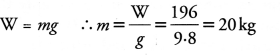Based on gravitation and newtons law of gravitation

Question 1.
State Newtons law of gravitation. Write the mathematical equation for Newton’s law of gravitation.
(CBSE 2010, 2011, 2014, 2015)
According to this law, the force of attraction between two particles or bodies is

1. directly proportional to the product of their masses and
2. inversely proportional to the square of the distance between these particles or bodies

Relation between gravitational force between two bodies and the distance between them.
Consider two bodies A and B having masses m1 and m2 respectively. Let the distance between these bodies be r (Figure 6).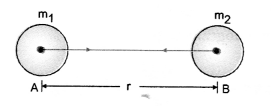According to the law of gravitation, the force of attraction (F) or force of gravitation between these bodies is directly proportional to (i) product of masses of bodies
i.e.,                                           F ∝ m1m2                                                                      …(1)
and (ii) inversely proportional to square of distance between bodies.Eqn. (3) gives the magnitude of gravitational force between two interacting bodies of masses m1 and m2 separated by a distance V.

Question 2.
Why is Newton’s law of gravitation known as universal law of gravitation ? (CBSE 2012)
This is because this law is applicable to all the objects or particles in the universe.

Question 3.
Why is G called universal constant ? (CBSE 2010, 2011, 2013, 2014)
Because the value of G = 6.673 x 10-11 Nm2 kg-2 is same at all positions in the universe.

Question 4.
How does the gravitational force between two bodies change if the distance between them is tripled ? Explain. (CBSE 2010, 2011)
Gravitational force between two bodies is given by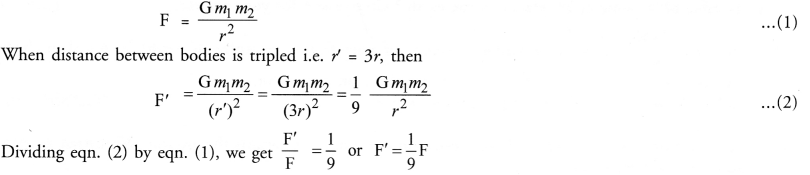Thus, the gravitational force between the bodies becomes 1/9 th times its original value.

Question 5.
State the source of centripetal force that a planet requires to revolve around the sun. On what factors does that force depend ? Suppose this force suddenly becomes zero, then in which direction will the planet begin to move if no other celestial body affects it ?
Gravitational force of attraction between the sun and the earth is the source of the centripetal force that a planet requires to revolve around the sun.
It depends on

1. the masses of the sun and the planet and
2. distance between the sun and the planet. If this force suddenly becomes zero, the planet begins to move in a straight line along the tangent at the point on the circular path of the planet around the sun because of inertia of motion.

Question 6.
Mention any two phenomena which will successfully be explained on the basis of Universal law of gravitation.

1. Existence of earth’s atmosphere
2. Revolution of planets around the sun.

Question 7.
The moon is acted by the gravitational pull of earth, still it does not fall onto the earth. Explain, why ?
(CBSE 2012, 2013)
The gravitational pull of earth on the moon provides the necessary force to the moon to move around the earth. Therefore, moon does not fall on the earth.

Question 8.
What is the cause of ocean tides ?

Or

Name the type of force which is responsible for the formation of tides in the sea. (CBSE 2014)
The earth exerts a large gravitational pull on the moon. According to Newton’s third law of motion, moon also exerts the same large gravitational pull on the earth. This large gravitational pull or force on the earth . due to moon causes ocean tides.

Question 9.
An apple attracts the earth and the earth also attracts the apple towards its centre. Then, why only apple falls towards the earth but earth is not moved towards the apple ?
(CBSE Sample Paper; CBSE 2010, 2011)

Or

A stone and the earth attract each other with an equal and opposite force, why then we see only the stone falling towards the earth, but not the earth rising towards the stone. (CBSE 2013)
The gravitational forces acting on both the apple and the earth (or a stone and earth) are equal in accelaration produced in the apple or stone is very large and the acceleration produced in the earth magnitude. Since, the mass of the apple or stone is very small as compared to the mass of the earth, so the
is negligible. That is why, the motion of the earth is not noticed.

Based on free fall and acceleration due to gravity

Question 10.
Two boys drop a sheet of a paper from the top of the tower. One boy dropped his sheet of paper as such
but the second boy dropped it by changing it in the form of a ball. Which sheet of paper reaches the ground first and why ? Assume that air resistance is not negligible. (CBSE 2010, 2014)
When a body falls through air, the air resistance or force of friction due to air opposes its motion. Since the surface area of sheet of paper is more than the surface area of the paper when it is in the form of a ball. Thus, the opposition offered by air to the paper sheet is more than the opposition offered to the ball shaped sheet. Hence, the sheet changed into the ball shape reaches the ground earlier than the paper sheet.

Question 11.
Study the diagrams below.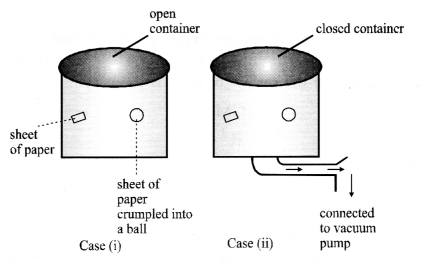A sheet of paper and a crumpled paper are thrown down from the same height and time is noted. Answer the following questions :
(a) Will the time taken to reach the bottom of the container be the same for the sheet of paper and crumpled paper ? Why ? Explain giving reason.
(b) What will happen in case (ii) ?
(a) Since the area of a sheet of paper is more than the area of the paper crumpled into a small, therefore, a sheet of paper will experience a large opposing force due to air than the ball, while falling down. Hence, a sheet of paper falls slower than one that is crumpled into a ball.
(b) Both will take same time to reach the bottom as there is no air resistance.

Question 12.
Derive an expression for the acceleration due to gravity. (CBSE 2010, 2011, 2012, 2013, 2014)

Or

How is the value of ‘g’ on the earth related to the mass of the earth and its radius. Derive the expression.
(CBSE 2015)
Consider a body of mass m near the surface of the earth (Figure 11). The force acting on the body is the gravitational force of the earth. The magnitude of the gravitational force acting on the body due to the earth is givenwhere, M = mass of the earth
R = radius of the earth
[Here, height of the body from the surface of the earth is neglected as compared to the radius of the earth because R = 6400 km is very large.]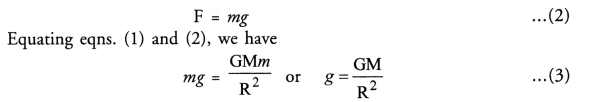This gravitational force (F) produces acceleration equal to ‘g in the body of mass m. So according to Newton’s second law of motion, which is the expression for the acceleration due to gravity.
Eqn. (3) shows that acceleration due to gravity does not depend on the mass of the object or body. Thus, all objects hollow or solid, big or small should fall at the same rate.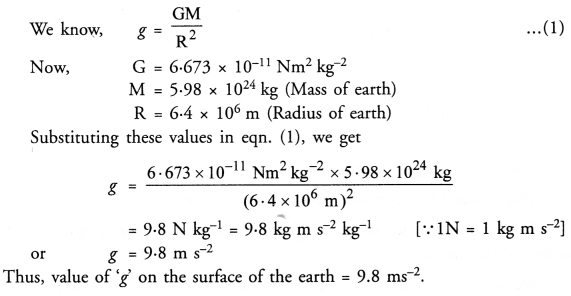Question 13.
Suppose a planet exists whose mass and radius both are half of those of earth. Calculate acceleration due to gravity on the surface of this planet. lg on the surface of the earth = 9.8 m s-2. (CBSE  2010, 2012)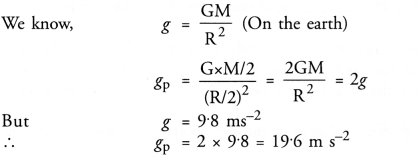Question 14.
What is the difference between ‘g and ‘G’ ?

 Acceleration due to gravity (g) Universal gravitational constant (G) 1. The acceleration produced in a body falling freely under the action of gravitational pull of the earth is known as acceleration due to gravity. The gravitational force between two bodies of unit masses separated by a unit distance is known as universal gravitational constant. 2. The value of ‘g’ is different at different points on the earth. The value of ‘G’ is same at every point on the earth. 3. The value of ‘g’ decreases as we go higher from the surface of the earth or as we go deep into the earth. The value of ‘G’ does not change with height and depth from the surface of the earth. 4. The value of ‘g’ at the centre of the earth is zero. The value of ‘G’ is not zero at the centre of the earth or anywhere else. 5. The value of is different on the surfaces of different heavenly bodies like the sun, the moon, the planets. The value of ‘G’ is same throughout the universe. 6. The value of ‘g’ on the surface of the earth is 9.8 m s-2 The value of G = 6.673 x10-11 Nm2 kg-2, throughout the universe.

Question 15.
Why is the value of lg variable on the surface of earth ?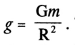The earth is oval shaped. The distance (R) of equator of earth from the centre of earth is greater than the distance of poles from the centre of earth. Hence, value of ‘g is different at equator and poles.

Question 16.
Give reasons :

1. The mass is constant everywhere whereas weight keeps changing.
2. The value of ‘g’ keeps changing as we move away from the earth whereas value of ‘G’ remains constant all over the universe.

1. Mass is a quantity of matter contained in a body and remains constant everywhere. Weight = mg. Since value of ‘g’ changes from place to place, so weight keeps on changing from one place to another place.
2. The value of ‘g with height is given bywhere h is the height from the surface of earth. As h increases, value of ‘g’ decreases. On the other hand, G is universal constant and its value remains constant all over the universe.

Question 17.
Where is the acceleration due to gravity minimum and maximum on the surface of the earth ? (CBSE 2015)
Acceleration due to gravity on the surface of earth is given bySince equatorial radius (RE) is greater than the polar radius (RP) of the earth, therefore, acceleration due to gravity is minimum at the equator and maximum at the poles.

Based on Mass and weight of a body

Question 18.
State the factors on which weight of a body depends. (CBSE 2012)
Since, weight, W = mg. So weight of a body depends on

1. mass of the body and
2. acceleration due to gravity (g) at the given place.

Question 19.
Why is the weight of a body less at equator than at poles ? (CBSE 2012, 2013, 2014)
Weight, W = mg. Since the value of ‘g’ is less at equator than at poles, so weight of a body is less at equator than at poles.

Question 20.
Why is the weight of a man less on the surface of the moon than on the surface of the earth ?
W = mg. Since value of ‘g’ on the moon is 1/6 times the value of ‘g’ on the earth. So weight of a body on moon is 1/6 times the weight of the body on earth.

Question 21.
Why is the weight of an object less on a mountain than at sea level ?
Since value of g decreases as we go higher and higher, so weight (mg) of the object on the mountain is less than at the sea level.

Question 22.
Why is the weight of a body zero at the centre of the earth ?
W = mg. Since g = 0 at centre of the earth.
∴ W = 0.

Question 23.
There are two kinds of balances i.e., a beam balance and a spring balance. If both the balances give the same measure of a given body on the surface of the earth, will they give the same measure on the surface of the moon ? Explain.
Beam balance measures the mass of a body. Since mass of a body remains constant so the beam balance will give the same measures on the surface of the earth and on the surface of the moon.
On the other hand, spring balance measures the weight of a body. Weight of a body W = mg. It means, weight of a body depends upon the value of ‘g’. Since value of ‘g on the moon = 1/6 times the value of ‘g’ on the earth, so the spring balance shows 1/6 th times the weight of the body on the earth at the surface of the moon.

Question 24.
A bag of sugar weighs W at some place on the equator. Will it weigh same or more or less when taken to Antarctica ? Explain.Equatorial radius is more than the polar radius, so the value of ‘g’ at pole is more than the value of ‘g’ at the equator. Hence, the sugar bag will weigh more at Antarctica (or poles).

Question 25.
A body of mass 10 kg is taken to the centre of earth. What will be its mass and weight there ?
Mass remains the same. That is, mass of body at the centre of earth = 10 kg.
Weight of body at the centre of earth, W = mg = 10 x 0 = 0 (∴ g at the centre of earth = 0).

Question 26.
Gravitational force on an imaginary planet is 6 times stronger than the gravitational force of the earth. Determine the value of acceleration due to gravity and weight of an object of mass 50 kg on that planet.
mgp = 6 mge
∴ gp = 6 ge = 6 x 9.8 = 58.8 ms-2
Weight of object on planet = mgp = 50 x 58.8 = 2940 N.

Question 27.
Find the weight of a 80 kg man on the surface of moon ? What should be his mass on the earth and on the moon ? (ge = 9.8 m/s2, gm = 1.63 m/s2).
Weight of man on moon = mgm = 80 x 1.63 = 130.4 N
Mass of an object does not change, when taken to moon from earth. Therefore, mass of man on earth and moon is same i. e., 80 kg.

Question 28.
An object has mass 1 kg and weight 1.67 N on moon. Calculate its weight and mass on earth (ge =10 m s-2).
Mass of object on earth = mass of object on moon = 1kg.
Weight of object on moon = (1/6) x weight of object on earth
Weight of object on earth = 6 Wm = 6 x 1.67 = 10.02 N

Question 29.
Find the weight of an object at a height 6400 km above the earth’s surface. The weight of the object at the surface of earth is 20 N and radius of earth is 6400 km.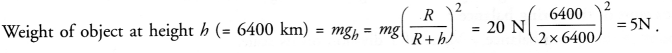Question 30.
A force of 20 N acts upon a body of weight 9.8 N. What is the mass of the body and how much is its acceleration ( g = 9.8 m s-2)?
W = mg =9.8 NQuestion 1.
(a) State Universal law of gravitation. Write the expression for the gravitational force between the earth and an object lying on the surface of the earth.
(b) Gravitational force plays an important role in nature. Justify this statement.

Or

State universal law of gravitation. Derive an expression for gravitational force between two bodies.
(CBSE 2012)
Definition of universal gravitational constant (G)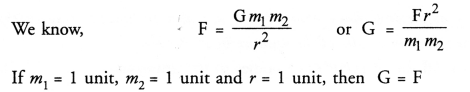If m1 = 1 unit, m2 = 1 unit and r = 1 unit, then G = F Thus, universal gravitational constant (G) is defined as the force of attraction between two bodies of unit masses separated by a unit distance.
Units of universal law of gravitation:Characteristics of Gravitational force

1. Gravitational force between two bodies or objects does not need any contact between them. It means, gravitational force is action at a distance.
2. Gravitational force between two bodies varies inversely proportional to the square of the distance between them. Hence, gravitational force is an inverse square force.
3. The gravitational forces between two bodies or objects form an action-reaction pair. If object A attracts object B with a force F1 and the object B attracts object A with a force F2 (Figure 7), then
4. Gravitational force between two small bodies is very small. On the other hand, gravitational force between two large bodies (say the sun and the earth) is large.
5. Gravitational force between two objects is always attractive in nature.

Question 2.
What do you understand by free fall ? Derive an expression for the acceleration due to gravity. Write the factors on which “acceleration due to gravity” depends.
Definition of Free Fall
The falling body on which only force of gravitation of the earth acts is known as freely falling body and such fall of a body is known as free fall. A freely falling body has constant acceleration equal to acceleration due to gravity (g), irrespective of its mass.Thus, all bodies irrespective of their masses fall down with constant acceleration.
Motion of a freely falling body is uniformly accelerated motion.
Consider a body of mass m near the surface of the earth (Figure 11). The force acting on the body is the gravitational force of the earth. The magnitude of the gravitational force acting on the body due to the earth is givenwhere, M = mass of the earth
R = radius of the earth
[Here, height of the body from the surface of the earth is neglected as compared to the radius of the earth because R = 6400 km is very large.]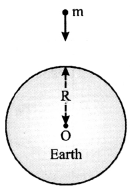This gravitational force (F) produces acceleration equal to ‘g in the body of mass m. So according to Newton’s second law of motion, which is the expression for the acceleration due to gravity.
Eqn. (3) shows that acceleration due to gravity does not depend on the mass of the object or body. Thus, all objects hollow or solid, big or small should fall at the same rate.

Question 3.
Define the terms “mass” and “weight”. Write their SI units. Distinguish between mass and weight.